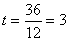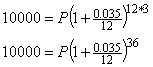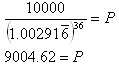Formula
Example 2

Suppose that you plan to need \$10,000 in thirty-six months' time when your child starts attending college. You want to invest in an instrument yielding 3.5% interest, compounded monthly. How much should you invest?

Step 1. Identify the variables.

A is the ending amount, in this case \$10,000.

P is the beginning amount, this is what we are trying to determine.

r is the interest rate written in the form of a decimal, in this case 0.035.

t is the time in years, in this caseyears.

n is the number of times compounded per year, in this case it is being compounded monthly and therefore n = 12.

Step 2. Substitute the variables in to the formula.Step 3. Calculate the formula, you may need a calculator.You need to invest about \$9004.62.